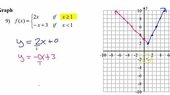# Use Graph To Determine Limit

• nycmathguy

#### nycmathguy

Summary:: Graphs and Limits

Use the graph to determine the limit of the piecewise function as x tends to 1.

Let me see.

lim of (-x + 3) as x-->1 from the left is 2.

lim of (2x) as x-->1 from the right is 2.

I can safely say that the limit of f(x) as x tends to 1 from the left and right simultaneously is 1.

The limit of f(x) is 1.

Correct?

#### Attachments

•th.jpeg-4.jpg
15.3 KB · Views: 71
Summary:: Graphs and Limits

lim of (-x + 3) as x-->1 from the left is 2.

lim of (2x) as x-->1 from the right is 2.

I can safely say that the limit of f(x) as x tends to 1 from the left and right simultaneously is 1.

The limit of f(x) is 1.

Correct?

Typo ?

##\ ##

•nycmathguy
Typo ?

##\ ##

Yes, big time typo. The limit is clearly 2 not 1. I was rushing through my first reply. Thank you for pointing out my typo. I will repost.

•Delta2

Use the graph to determine the limit of the piecewise function as x tends to 1.

Let me see.

lim of (-x + 3) as x-->1 from the left is 2.

lim of (2x) as x-->1 from the right is 2.

I can safely say that the limit of f(x) as x tends to 1 from the left and right simultaneously is 2.

The limit of f(x) is 2.

P. S. Having fun with calculus so far. Hoping the excitement does not run out.

•BvU and Delta2